# The determinant

The determinant of the unit matrix equals 7. Check how many rows the A matrix contains.

Result

n =  7

#### Solution:Leave us a comment of example and its solution (i.e. if it is still somewhat unclear...):

Showing 0 comments:Be the first to comment!## Next similar examples:

1. Inverse matrix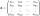Find how many times is the larger determinant is the matrix A, which equals 9 as the determinant of its inverse matrix.
2. The inverseThe inverse matrix for matrix A has a determinant value of 0.333. What value has a determinant of the matrix A?
3. Inverse matrix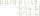Find out inverse by Gauss elimination or by reduction method. A=[2/3. 1 -3. 1/3]
4. RootsDetermine the quadratic equation absolute coefficient q, that the equation has a real double root and the root x calculate: ?
5. Balls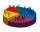We have n identical balls (numbered 1-n) is selected without replacement. Determine 1) The probability that at least one tensile strength number coincides with the number of balls? 2) Determine the mean and variance of the number of balls, which coincides.
6. AS sequenceIn an arithmetic sequence is given the difference d = -3 and a71 = 455. a) Determine the value of a62 b) Determine the sum of 71 members.
7. The confectionery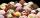The confectionery sold 5 kinds of ice cream. In how many ways can I buy 3 kinds if order of ice creams does not matter?
8. TrinityHow many different triads can be selected from the group 43 students?
9. Linsys2Solve two equations with two unknowns: 400x+120y=147.2 350x+200y=144
10. Derivation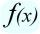Exists a function whose derivation is the same function?
11. Theorem proveWe want to prove the sentense: If the natural number n is divisible by six, then n is divisible by three. From what assumption we started?
12. Sequence 3Write the first 5 members of an arithmetic sequence: a4=-35, a11=-105.
13. Euclidean distanceCalculate the Euclidean distance between shops A, B and C, where: A 45 0.05 B 60 0.05 C 52 0.09 Wherein the first figure is the weight in grams of bread and second figure is price in USD.
14. Median and modusRadka made 50 throws with a dice. The table saw fit individual dice's wall frequency: Wall Number: 1 2 3 4 5 6 frequency: 8 7 5 11 6 13 Calculate the modus and median of the wall numbers that Radka fell.
15. CalculationHow much is sum of square root of six and the square root of 225?
16. 75th percentile (quartille Q3)Find 75th percentile for 30,42,42,46,46,46,50,50,54
17. Calculation of CNCalculate: ?